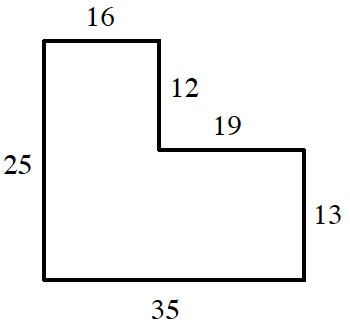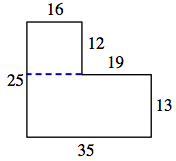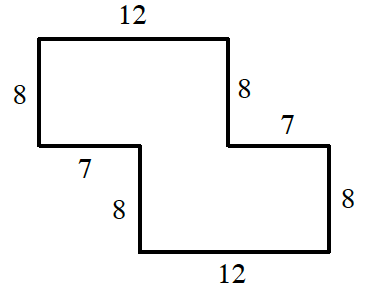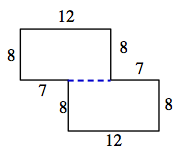### Home > CCA > Chapter A > Lesson A.1.1 > ProblemA-8

A-8.

Copy the following figures onto your paper. Then find the area and perimeter of each shape, assuming that all corners are right angles. Be sure to show all of your work.

1.To find the area, separate the shape into two boxes, find the area of the two sections, and then add them together for the total area.$16 · 12 = 192$
$13 · 35 = 455$
$\text{Area} = 192 + 455$

$\text{Area} = 647$

To find the perimeter (the distance around a shape) add up all of the sides of the shape.

$\text{perimeter} = 16 + 12 + 19 + 13 + 35 + 25$

$\text{perimeter} = 120$

1.Follow the same strategy outlined in part (a).